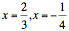Te Kete Ipurangi
Communities
Schools

## Senior Secondary navigation

#### Section menu

Achievement objectives

What has changed:

# Achievement objective M7-7

In a range of meaningful contexts, students will be engaged in thinking mathematically and statistically. They will solve problems and model situations that require them to:

• form and use linear, quadratic, and simple trigonometric equations.

## Indicators

• Solves problems that can be modelled by a combination of linear or quadratics, simple trigonometric equations and interprets solutions in context.
• Uses completing the square and quadratic formula for solving quadratic equations.
• Links discriminant with nature of the solutions of a quadratic equation.
• Demonstrates understanding of the relationship between an equation and its solutions, for example, no, one, or two solutions for quadratic equations, multiple solutions for trigonometric equations.
• Makes links with trigonometric relationships M7-4, algebraic manipulation M7-6, and simultaneous equations M7-8.
• See key mathematical ideas on NZmaths.

## Progression

M7-7 links from NA6-5 and to M8-7, M8-9.

## Possible context elaborations

• Solving equations such as Asin(Bx) = C in degrees.
• A quadratic equation has given solutions; find the equation in the general form ax^2 + bx + c = 0.
• For what values of a does the equation x^2 = 6x + a have real solutions?
• Chris answered a multiple-choice test with 50 questions. Correct answers score 4 points and every incorrect answer loses one mark. She misses out questions she is not sure of. Her total mark is 47. Find the least and the greatest number of questions she could have answered.
• What is the relationship between the solutions of x^2 + bx + c = 0 and cx^2 + bx + 1 = 0?
• Activity: Coffee costs
• Activity: Skid marks
• Automobile pistons: Investigate the position of the piston in an automobile engine in relationship to the crankshaft. What graph does this represent?
• Triathlon: Andrea Hewitt is racing in a triathlon and is doing the cycle leg. What is the vertical displacement of her knee as she rides along the road? What graph does this represent?
• Windscreen wipers: Calculate the area (using radians) covered by one windscreen wiper of a car.

## Assessment for qualifications

NCEA achievement standards at level 1, 2 and 3 have been aligned to the New Zealand Curriculum. Please ensure that you are using the correct version of the standards by going to the NZQA website.

The following achievement standard(s) could assess learning outcomes from this AO:

Last updated September 16, 2018